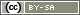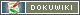[[diagram()]]

# diagram()

`string diagram(string Typ, string Variable, [string options])`

The function `diagram()` evaluates all data collected so far and displays a diagram directly in the questionnaire. The function returns HTML code which can be displayed e.g. using `html()` or via wildcard.

• Typ
Two types of diagrams are available (see below for details):
• `'bar`' – Column chart
• `'percent`' – Bar graph for multiple answers
• Variable
At least one valid variable identifier must be specified as variable, as listed in the Variables overview. Multiple variables are passed as separate arguments.
• Options
Depending on the chart type, other options are available:
• 'value=<N>'
Specifying a value (for type 'percent')
• 'width=<N>'
Specify how wide the diagram should be, in pixels
• 'height=<N>'
Indication of how high the diagram should be, in pixels

Note: Only completely filled out questionnaires are evaluated for the diagram (see Variable FINISHED).

Note: Please also see the notes on function `statistic()`. There, it is explained in detail which data sets are included in the statistics.

Note: The data from the current interview will only appear in the statistics after clicking “Next” twice. The procedure is as follows: First the question is given. After clicking on “Next” the answers are read in (but not yet saved), then the next page is built and only then the data is saved. On the page that directly follows a question, the information about the question is therefore not yet included in the statistics.

## Column Chart

The column chart (chart type `'bar`') shows as labels the numerical codes of the answers. The frequency of each answer (given at least once so far) is displayed as a column.

As Variable a variable from the dataset has to be specified (see Variables overview).

In the following example it is assumed that question “AB01” is a simple selection:

```html(
diagram('bar', 'AB01')
);```

## Bar Graph for Multiple Responses

The bar chart for multiple answers (chart type `'percent`') shows the answer alternatives as labels. The bars show how often a certain value (e.g. `2` as code for “checked”) was selected in different questions. It makes sense to use this type of diagram for a multiple selection if you offer the variables of the different items in the multiple selection.

The value to be counted must be passed as an option: Z.B. 'value=2', to show how many times the option was selected in a multiple selection.

```html(
diagram('percent', 'TR01_01', 'TR01_02', 'value=2', 'width=200')
);```